# How to Calculate and Solve for Rate of Homogeneous Reaction | Corrosion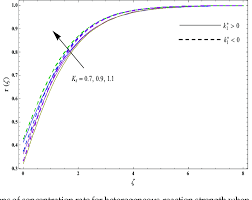The image above represents rate of homogeneous reaction.

To compute for rate of homogeneous reaction, two essential parameters are needed and these parameters are dn/dt and Stoichiometric Number (v).

The formula for calculating rate of homogeneous reaction:

Vr = dn/dt / v

Where:

Vr = Rate of Homogeneous Reaction
dn/dt = dn/dt
v = Stoichiometric Number

Let’s solve an example;
Find the rate of homogeneous reaction when the dn/dt is 24 and the stoichiometric number is 12.

This implies that;

dn/dt = dn/dt = 24
v = Stoichiometric Number = 12

Vr = dn/dt / v
Vr = 24 / 12
Vr = 2

Therefore, the rate of homogeneous reaction is 2.

Calculating the dn/dt when the Rate of Homogeneous Reaction and the Stoichiometric Number is Given.

dn/dt = Vr x v

Where;

dn/dt = dn/dt
Vr = Rate of Homogeneous Reaction
v = Stoichiometric Number

Let’s solve an example;
Find the dn/dt when the rate of homogeneous reaction is 18 and the stoichiometric number is 4.

This implies that;

Vr = Rate of Homogeneous Reaction = 18
v = Stoichiometric Number = 4

dn/dt = Vr x v
dn/dt = 18 x 4
dn/dt = 72

Therefore, the dn/dt is 72.

Calculating the Stoichiometric Number when the Rate of Homogeneous Reaction and the dn/dt is Given.

v = dn/dt / Vr

Where;

v = Stoichiometric Number
Vr = Rate of Homogeneous Reaction
dn/dt = dn/dt

Let’s solve an example;
Find the stoichiometric number when the rate of homogeneous reaction is 12 and the dn/dt is 36.

This implies that;

Vr = Rate of Homogeneous Reaction = 12
dn/dt = dn/dt = 36

v = dn/dt / Vr
v = 36 / 12
v = 3

Therefore, the stoichiometric number is 3.

Nickzom Calculator – The Calculator Encyclopedia is capable of calculating the rate of homogeneous reaction.

To get the answer and workings of the rate of homogeneous reaction using the Nickzom Calculator – The Calculator Encyclopedia. First, you need to obtain the app.

You can get this app via any of these means:

To get access to the professional version via web, you need to register and subscribe for NGN 2,000 per annum to have utter access to all functionalities.
You can also try the demo version via https://www.nickzom.org/calculator

Apple (Paid) – https://itunes.apple.com/us/app/nickzom-calculator/id1331162702?mt=8
Once, you have obtained the calculator encyclopedia app, proceed to the Calculator Map, then click on Materials and Metallurgical under Engineering.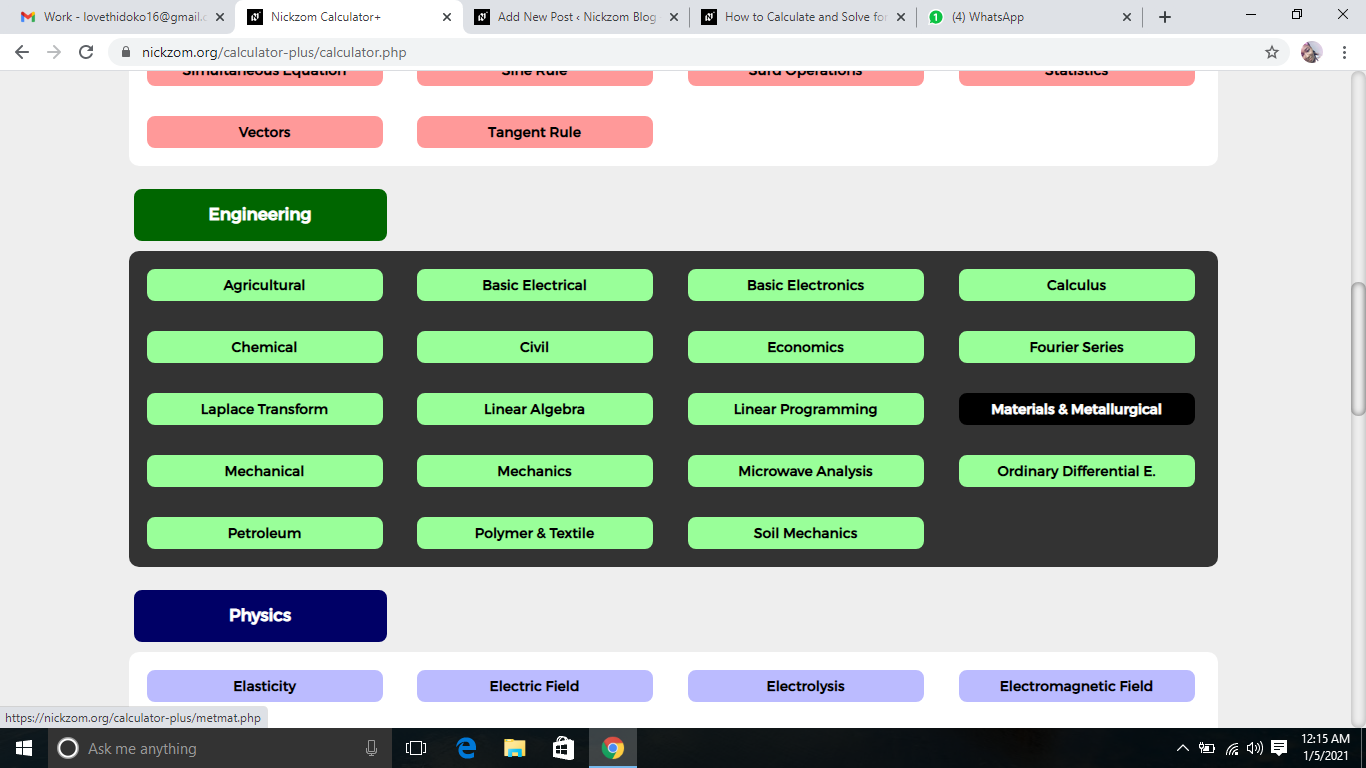Now, Click on Corrosion under Materials and Metallurgical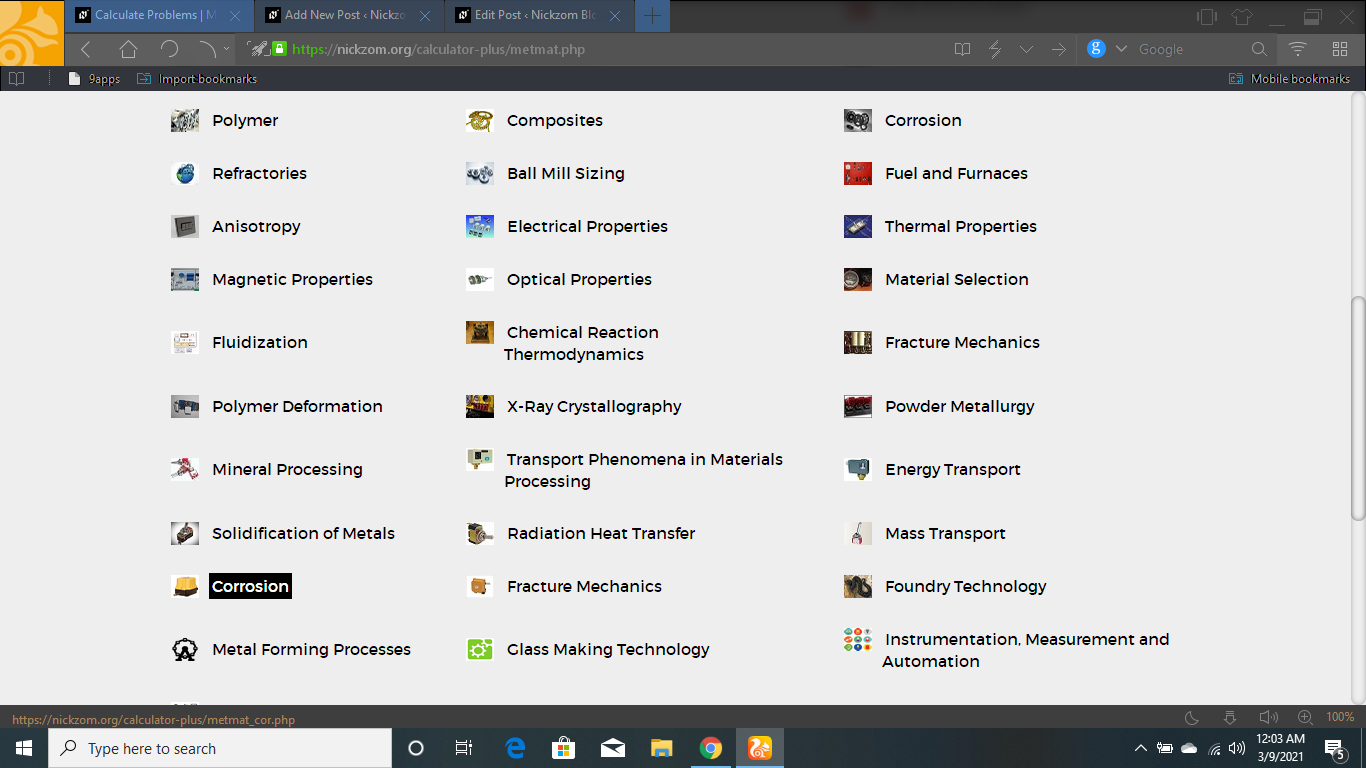Now, Click on Rate of Homogeneous Reaction under Corrosion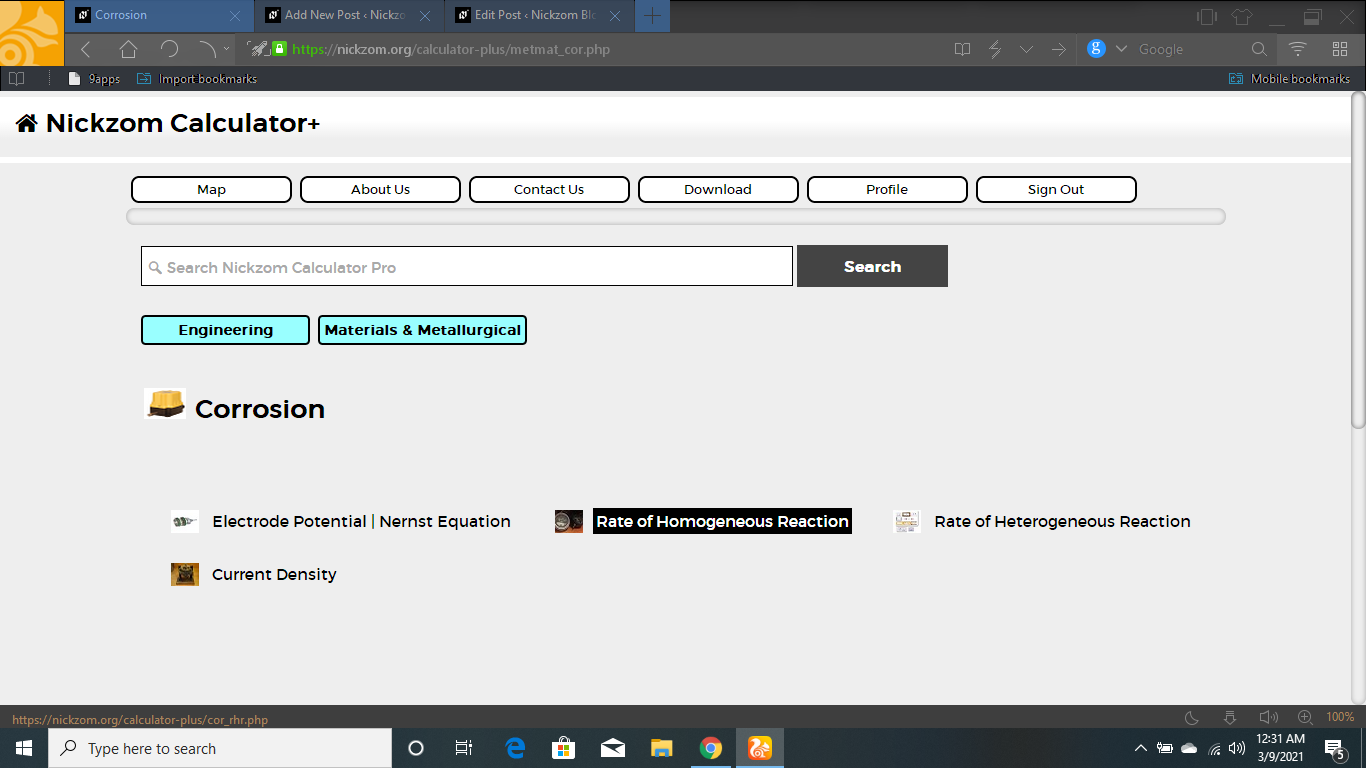The screenshot below displays the page or activity to enter your values, to get the answer for the rate of homogeneous reaction according to the respective parameters which is the dn/dt and Stoichiometric Number (v).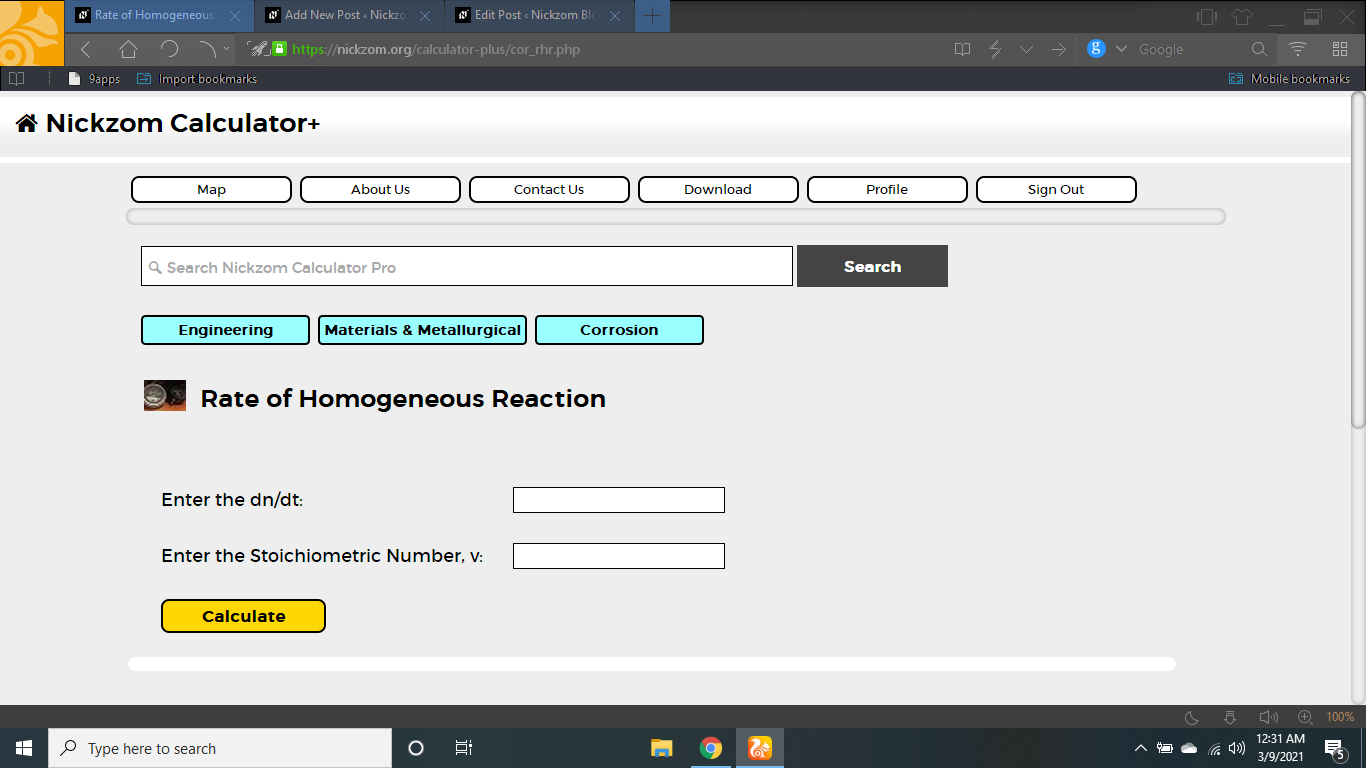Now, enter the value appropriately and accordingly for the parameter as required by the dn/dt is 24 and Stoichiometric Number (v) is 12.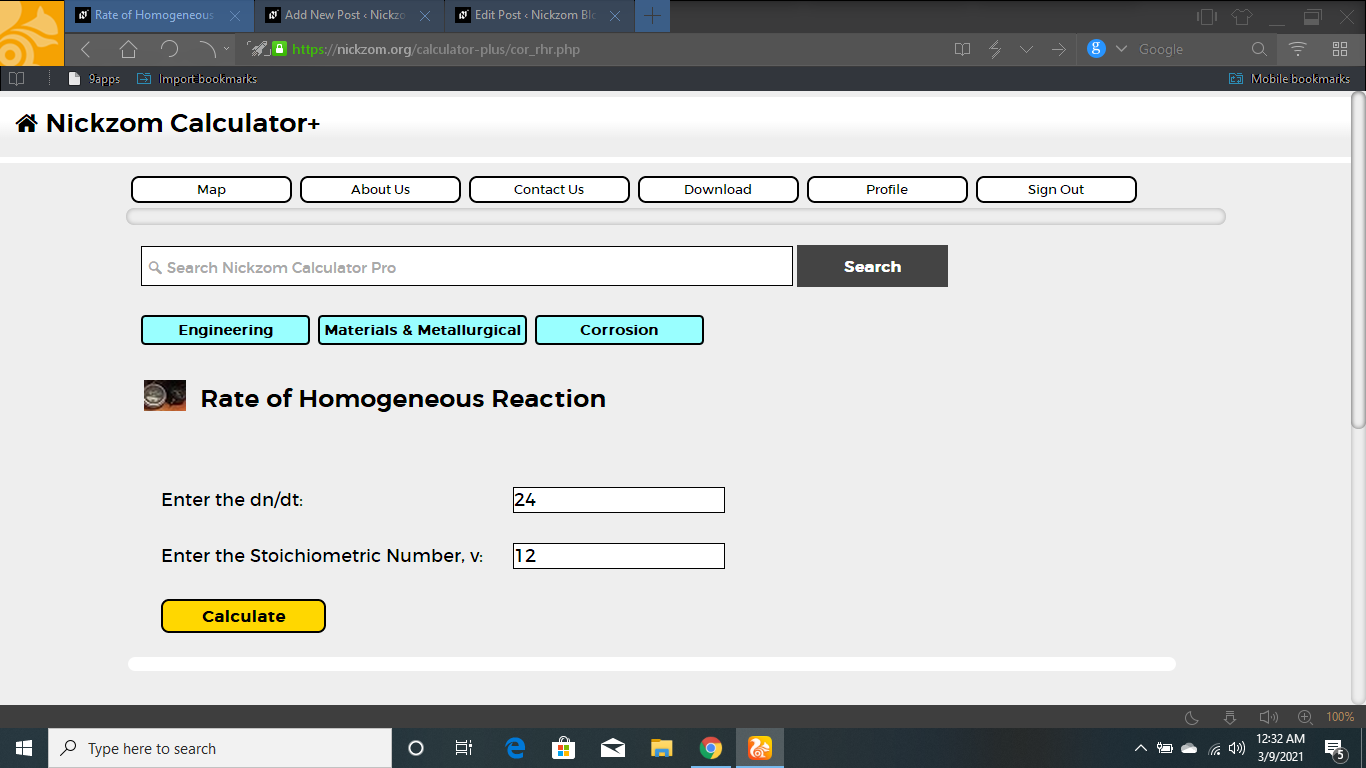Finally, Click on Calculate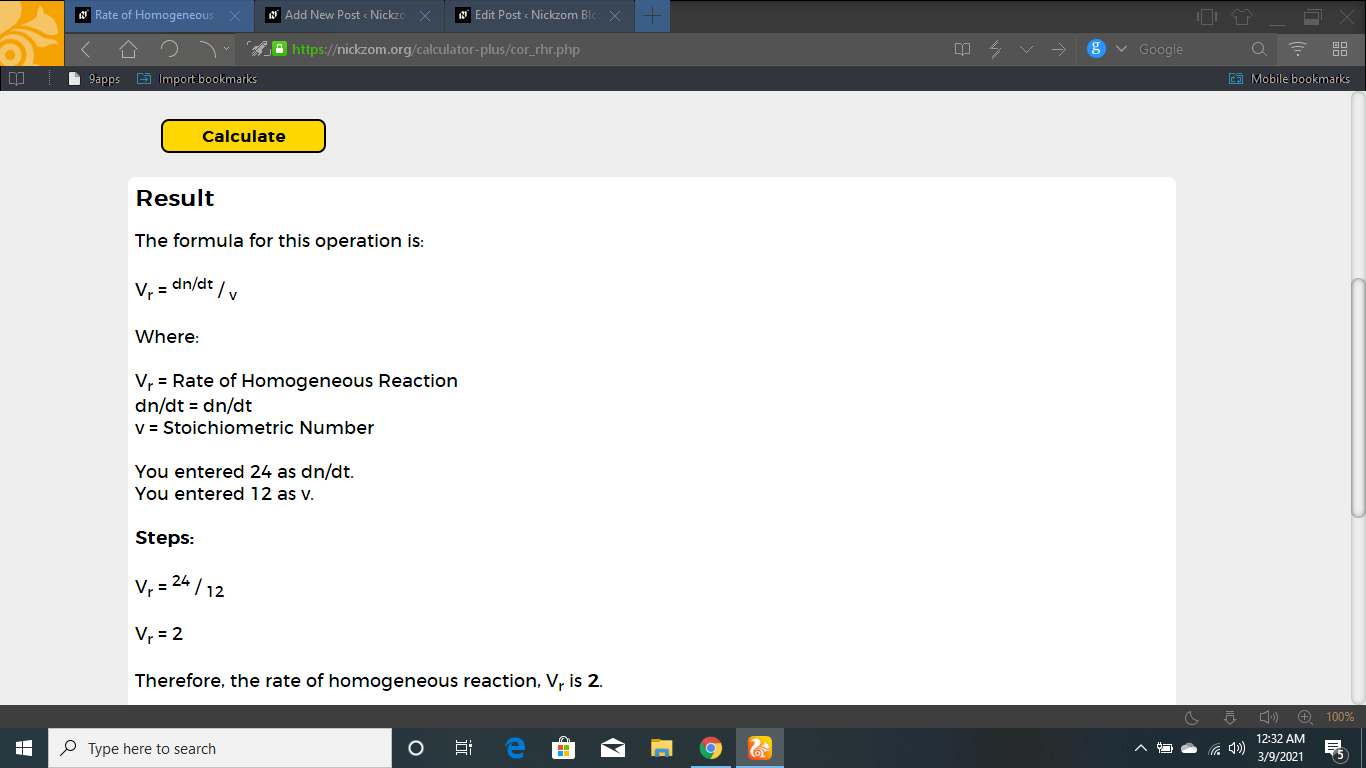As you can see from the screenshot above, Nickzom Calculator– The Calculator Encyclopedia solves for the rate of homogeneous reaction and presents the formula, workings and steps too.